## Excel CHOOSE function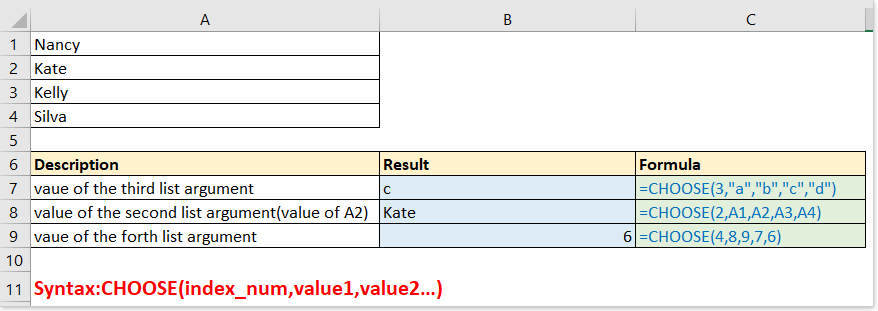Description

Syntax and Arguments

Usage and Examples

### Description

The CHOOSE function returns a value from the list of value argument based on the given index number. For example, CHOOSE(3,”Apple”,”Peach”,”Orange”) returns Orange, the index number is 3, and Orange is the third value after index number in the function.

### syntax and Arguments

Formula syntax

 CHOOSE(index_num, value1, [value2], …)

Arguments

 Index_num: Required. A numeric value between 1-254 to indirect which argument value will be selected. if index_num is out of the scale between 1-254, CHOOSE returns the #VALUE! error value. If index_num is a decimal value, it will be rounded down to a whole number before formula being used. Value1: Required. The first value in the formula that can be choose. Value2: Optional. The second and up to 254th value from which to choose.

Value1, value2… can be numbers, texts, formulas, cell references, or defined name.

Return Value

The CHOOSE function returns a value from a list based on the given position.

### Usage and Examples

In this part, I list some simple but representative examples to explain the usage of the CHOOSE function.

Ex1 - Basic usage: using CHOOSE function alone to choose value from list argument

Formula1:

=CHOOSE(3,"a","b","c","d")

Return: c, which is the third argument after the index_num of 3 in the CHOOSE function.

Note: using double quotes surrounding the value if it is text.

Formula2:

=CHOOSE(2,A1,A2,A3,A4)

Return: Kate, the value of A2. Since the index_num is 2, and A2 is the second value in the CHOOSE function.

Formula3:

=CHOOSE(4,8,9,7,6)

Return: 6, the 4th list argument in the function.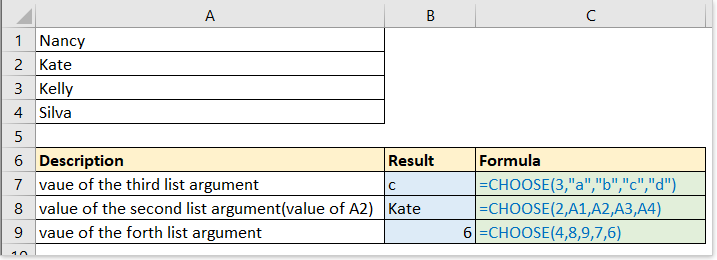Ex2 – Return different results based on multiple conditions

Supposing you have a list of deviations for each product which needed to be labeled based on conditions as below screenshot shown.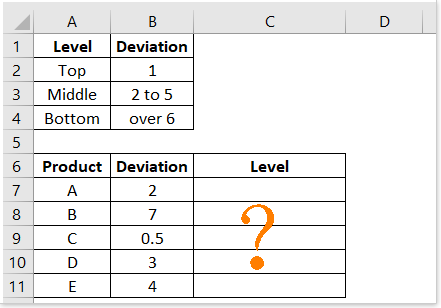Usually, you can use the IF function to handle, but here I introduce how to use the CHOOSE function to solve this problem easily

Formula:

=CHOOSE((B7>0)+(B7>1)+(B7>5),"Top","Middle","Bottom")

Explain:

(B7>0)+(B7>1)+(B7>5): the index_num, B7 is 2, which is bigger than 0 and 1 but less than 5, so we get the intermediate result:

=CHOOSE(True+Ture+False,"Top","Middle","Bottom")

As we know, True = 1, False = 0, so the formula can be viewed as :

=CHOOSE(1+1+0,"Top","Middle","Bottom")

then

=CHOOSE(2,"Top","Middle","Bottom")

Result: Middle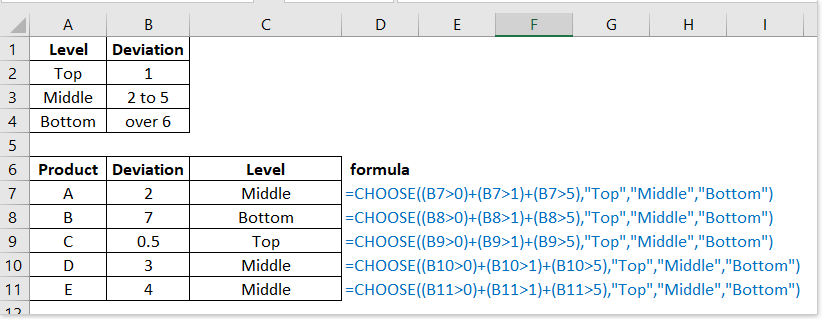Ex3 – Return different calculated results based on conditions

Supposing you need to calculate the rebates for each product based on the amount and price as below screenshot shown: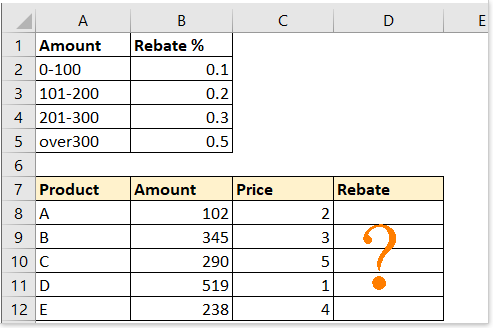Formula:

=CHOOSE((B8>0)+(B8>100)+(B8>200)+(B8>300),B8*C8*0.1,B8*C8*0.2,B8*C8*0.3,B8*C8*0.5)

Explain:

(B8>0)+(B8>100)+(B8>200)+(B8>300): index_number, B8 is 102, which is larger than 100 but less than 201, so in this part, it returns result shown as:

=CHOOSE(true+true+false+false,B8*C8*0.1,B8*C8*0.2,B8*C8*0.3,B8*C8*0.5)

=CHOOSE(1+1+0+0,B8*C8*0.1,B8*C8*0.2,B8*C8*0.3,B8*C8*0.5)

then

=CHOOSE(2,B8*C8*0.1,B8*C8*0.2,B8*C8*0.3,B8*C8*0.5)

B8*C8*0.1,B8*C8*0.2,B8*C8*0.3,B8*C8*0.5: the values from which to choose, rebate equals to price * amount * rebate percent, since here index_num is 2, it chooses B8*C8*0.2

Returns: 102*2*0.2=40.8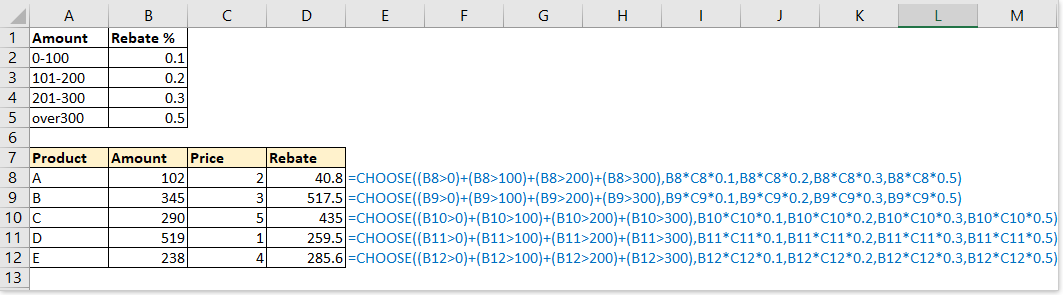Ex4 – Choose random from list

In Excel, sometimes, you may need to randomly choose a value from a given list, the CHOOSE function can solve this job.

Randomly choose one value from a list:

Formula:

=CHOOSE(RANDBETWEEN(1,5),\$D\$2,\$D\$3,\$D\$4,\$D\$5,\$D\$6)

Explain:

RANDBETWEEN(1,5): index_num, randomly obtain number between 1 to 5

\$D\$2,\$D\$3,\$D\$4,\$D\$5,\$D\$6: the list of values from which to choose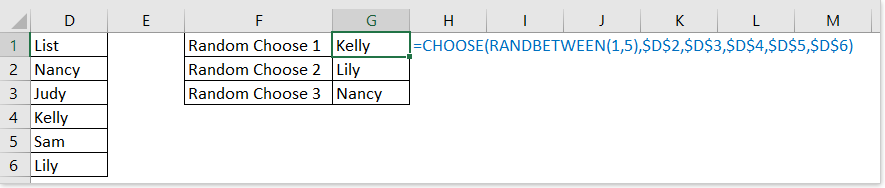Ex5 – Combine CHOOSE and VLOOKUP functions to return value in left column

Generally, we use the VLOOKUP function =VLOOKUP (value, table, col_index, [range_lookup]) to return a value based on a given value from a table range. But with the VLOOKUP function, it will return an error value while the return column is on the left of lookup column as below screenshot shown: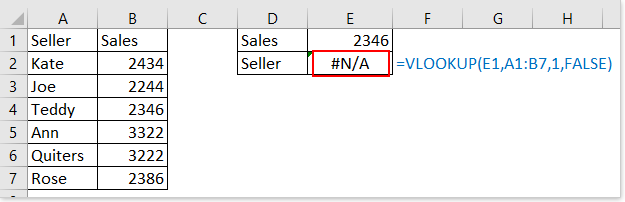In this case, you can combine the CHOOSE function with VLOOKUP function to solve the problem.

Formula:

=VLOOKUP(E1,CHOOSE({1,2},B1:B7,A1:A7),2,FALSE)

Explain:

CHOOSE({1,2},B1:B7,A1:A7): as table_range argument in the VLOOKUP function. {1,2} means to display 1 or 2 as index_num argument based on the col_num argument in VLOOKUP function. Here, the col_num in VLOOKUP function is 2, so the CHOOSE function display as CHOOSE(2, B1:B7,A1:A7), means to choose value from A1:A7.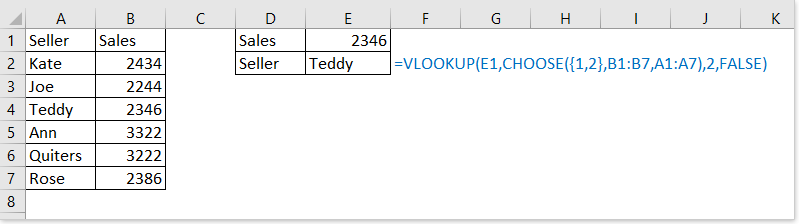Ex6 – Return weekday or month based on given date

With the CHOOSE function, you also can return the relative weekday and month based on a given date.

Formula 1: return weekday by a date

=CHOOSE(WEEKDAY(),"Sunday","Monday","Tuesday","Wednesday","Thursday","Friday","Saturday")

Explain:

WEEKDAY(): the index_num argument, to get the weekday number of the given date, for example, WEEKDAY(A5) returns 6, then the index_num argument is 6.

"Sunday","Monday","Tuesday","Wednesday","Thursday","Friday","Saturday": value list arguments, start with “Sunday” because weekday number “1” indicates “Sunday”.

Formula 2: return month by a date

=CHOOSE(MONTH(),"Jan","Feb","Mar","Apr","May","Jun","Jul","Aug","Sep","Oct","Nov","Dec")

Explain:

MONTH(): index_num argument, which get the month number from the given date, for example, MONTH(A5) returns 3.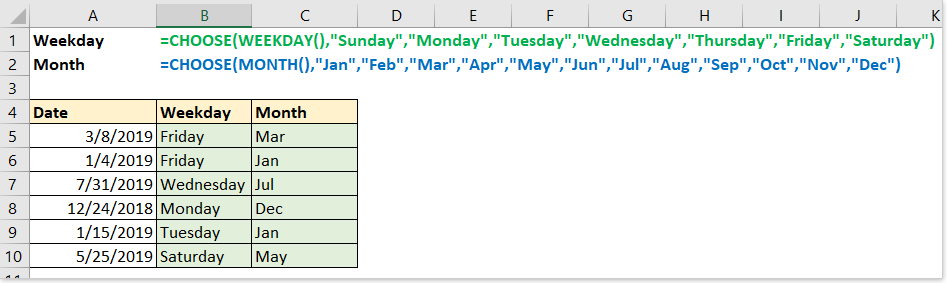In daily work, you may want to calculate the next workday or weekend based on today. Here the CHOOSE function also can do you a favor.

For instance, today is 12/20/2018, Thursday, now you need to obtain the next workday and weekend.

Formula 1: get the date of today

=TODAY()

Result: 12/20/2018

Formula 2: get the weekday number of today

=WEEKDAY(TODAY())

Result: 5 (while today is 12/20/2018)

The weekday number list as below screenshot shown: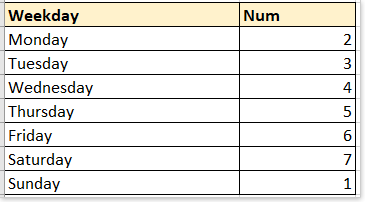Formula 3: get the next workday

=TODAY()+CHOOSE(WEEKDAY(TODAY()),1,1,1,1,1,3,2)

Explain:

Today(): return the current date

WEEKDAY(TODAY()): index_num argument in the CHOOSE function, get the weekday number of today, for example, Sunday is 1, Monday is 2…

1,1,1,1,1,3,2: value list argument in the CHOOSE function. For example, if weekday(today()) returns 1 (Sunday), it choose 1 form the list of values, then the whole formula changes to =Today()+1, which means to add 1 day to return next Monday. If weekday(today()) returns 6 (Friday), it chooses 3 from the list of values, because Friday is 3 days far from next Monday.

Result (while today is 12/20/2018):

=12/20/2018+CHOOSE(5,1,1,1,1,1,3,2)

=12/20/2018+1

=12/21/2018

Formula 4: get the next weekend day

=TODAY()+CHOOSE(WEEKDAY(TODAY()),6,5,4,3,2,1,1)

Explain:

6,5,4,3,2,1,1: value list argument in the CHOOSE function. For example, if weekday(today()) returns 1 (Sunday), it chooses 6 from the list of values, then the whole formula changes to =Today()+6, which means to add 6 days and return next Saturday.

Result:

=12/20/2018+CHOOSE(5,6,5,4,3,2,1,1)

=12/20/2018+2

=12/22/2018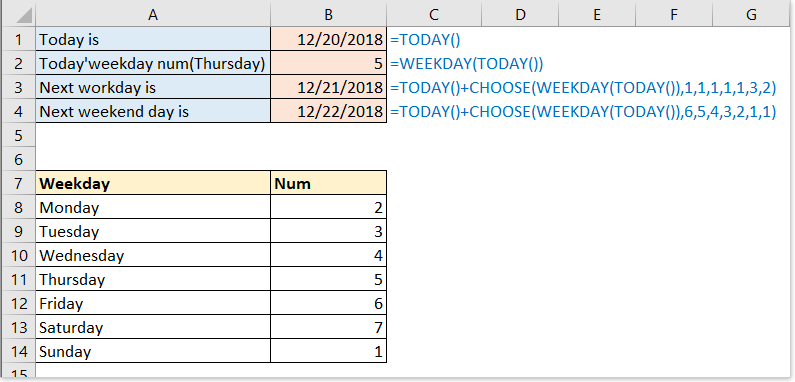### The Best Office Productivity Tools

#### Kutools for Excel - Helps You To Stand Out From Crowd

 Popular Features: Find, Highlight or Identify Duplicates  |  Delete Blank Rows  |  Combine Columns or Cells without Losing Data  |  Round without Formula ... Super VLookup: Multiple Criteria  |  Multiple Value  |  Across Multi-Sheets  |  Fuzzy Lookup... Adv. Drop-down List: Easy Drop Down List  |  Dependent Drop Down List  |  Multi-select Drop Down List... Column Manager: Add a Specific Number of Columns  |  Move Columns  |  Toggle Visibility Status of Hidden Columns  |  Compare Columns to Select Same & Different Cells ... Featured Features: Grid Focus  |  Design View  |  Big Formula Bar  |  Workbook & Sheet Manager | Resource Library (Auto Text)  |  Date Picker  |  Combine Worksheets  |  Encrypt/Decrypt Cells  |  Send Emails by List  |  Super Filter  |  Special Filter (filter bold/italic/strikethrough...) ... Top 15 Toolsets:  12 Text Tools (Add Text, Remove Characters ...)  |  50+ Chart Types (Gantt Chart ...)  |  40+ Practical Formulas (Calculate age based on birthday ...)  |  19 Insertion Tools (Insert QR Code, Insert Picture from Path ...)  |  12 Conversion Tools (Numbers to Words, Currency Conversion ...)  |  7 Merge & Split Tools (Advanced Combine Rows, Split Excel Cells ...)  |  ... and more

Kutools for Excel Boasts Over 300 Features, Ensuring That What You Need is Just A Click Away...#### Office Tab - Enable Tabbed Reading and Editing in Microsoft Office (include Excel)

• One second to switch between dozens of open documents!
• Reduce hundreds of mouse clicks for you every day, say goodbye to mouse hand.
• Increases your productivity by 50% when viewing and editing multiple documents.
• Brings Efficient Tabs to Office (include Excel), Just Like Chrome, Edge and Firefox.